Finite Difference Method using MATLAB

This section considers transient heat transfer and converts the partial differential equation to a set of ordinary differential equations, which are solved in MATLAB. This method is sometimes called the method of lines. We apply the method to the same problem solved with separation of variables. It represents heat transfer in a slab, which is insulated at x = 0 and whose temperature is kept at zero at x = a. The initial conditions are that the temperature is 1.0 everywhere.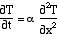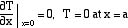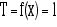The problem is sketched in the figure, along with the grid. The solution will be derived at each grid point, as a function of time.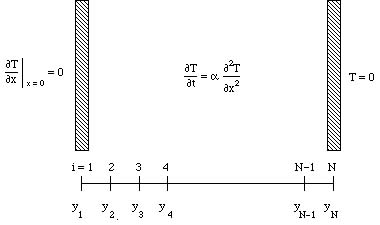Next we evaluate the differential equation at the grid points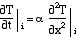We can evaluate the second derivative using the standard finite difference expression for second derivatives. The first term is really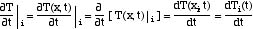Combining these equations gives the finite difference equation for the internal points.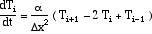(1)

At the boundary, x = 0, we also need to use a false boundary and write the boundary condition as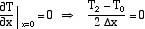We evaluate the differential equation at point 1 and insert the boundary values, T0 = T2, to get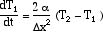(2)

For the outer boundary we use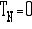(3)

If this equation is incorporated into the N-1-st equation we get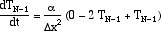(4)

Thus the problem requires solving Eq. (2) for point 1 and Eq. (1) for n = 2,...,N-2, and Eq. (4) for N­1. The initial conditions are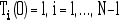In these equations there is only one independent variable, so they are ordinary differential equations.

Since they are first order, and the initial conditions for all variables are known, the problem is an initial value problem.

The MATLAB program ode45 integrates sets of differential equations using a 4-th order Runge-Kutta method. The calling sequence is

[t,y] = ode45('rhs',tspan,y0)

The term in quotes, 'rhs', is the name of the script which defines the problem. The tspan = [t0 tf], where t0 is the starting time, and tf is the ending time. y0 is the set of initial values. Let us use 5 intervals, or six points, or N-1 = 5. Then we need to set

y0 = [ 1 1 1 1 1]

We also need to prepare a script for the right-hand side of the problem. The differential equations are (for N = 6)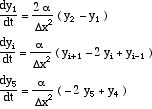The script is then

% saved as file rhs
function ydot=rhs(t,y)
global aa
nn=size(y)
n = nn(2)
ydot=zeros(n,1)
ydot(1) = 2*aa*(y(2)-y(1))
for i = 2:n-1
ydot(i) = aa*(y(i+1)-2*y(i)+y(i-1))
end
ydot(n) = aa*(-2*y(n) + y(n-1))

The script is written in a general fashion so that the number of points can be changed easily. In MATLAB, we would define 'aa' in the calling commands, and use global in the calling commands, too. The number of terms (which affects the value of aa we choose) is set by the number of initial conditions for y at time zero.

The problem is solved with a = 2 x 10-3 cm2/sec, L = 1 cm, and T0 = 1, and it is plotted in Figure 1 at different times. We see there the gradual change of the solution from the boundary, where the temperature has been set to zero, as time proceeds. We redo this solution with 11 points to verify that the solution is adequate. The plots in Figure 2 are similar to Figure 1 so that we conclude 6 points is sufficient for our purposes. If we wanted to see the effect of different initial conditions we have only to change them and rerun the problem. This solution, with 6 points, is adequate if we are interested in the time intervals plotted; if we are interested in smaller time intervals, though, we find the solution oscillates from node to node. In that case more points are needed.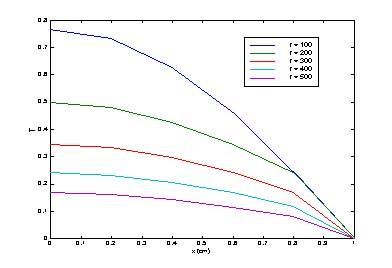Figure 1. Solution with 6 grid points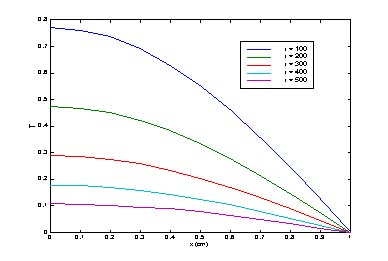Figure 2. Solution with 11 grid points

Next consider the diffusion problem.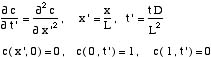The finite difference formulation of this problem is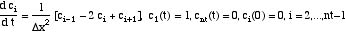The code is available. Solutions using 5, 9, and 17 grid points are shown in Figures 3-5. Five is not enough, but 17 grid points gives a good solution.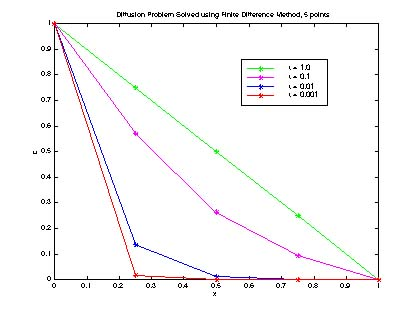Figure 3. Diffusion Problem solved with 5 Finite Difference Grid Points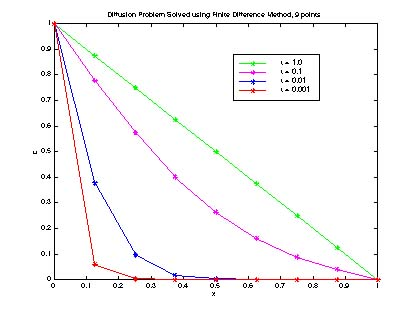Figure 4. Diffusion Problem solved with 9 Finite Difference Grid Points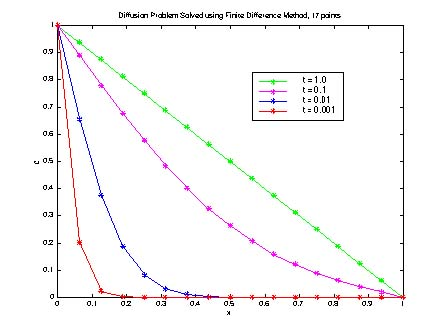Figure 5. Diffusion Problem solved with 17 Finite Difference Grid Points